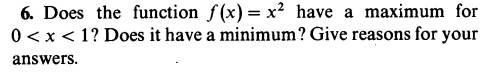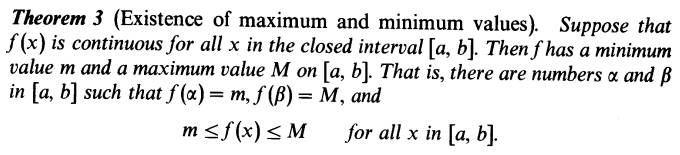# Maximum, minimum, and continuity

Karol

## Homework StatementTheorem 3 that i will give in the attempt at a solution talks about a closed interval, here it's open

## Homework Equations

Continuity:
$$\vert x-c \vert < \delta~\Rightarrow~\vert f(x)-f(c) \vert < \epsilon$$
$$\delta=\delta(c,\epsilon)$$

## The Attempt at a SolutionMentor

## Homework Statement

View attachment 204727
Theorem 3 that i will give in the attempt at a solution talks about a closed interval, here it's open

## Homework Equations

Continuity:
$$\vert x-c \vert < \delta~\Rightarrow~\vert f(x)-f(c) \vert < \epsilon$$
$$\delta=\delta(c,\epsilon)$$

## The Attempt at a Solution

View attachment 204728
Theorem 3 is no help here, since the interval for this problem is 0 < x < 1. The problem is fairly simple -- you shouldn't need to invoke a theorem to answer it.

Karol
The derivative 2x is horizontal at 0, so there is a minimum or maximum. the fact that there isn't any other zero derivative proves there isn't another bending and it's rising, so the maximum is at 1

dgambh
Remember ##0 < x < 1##. Also you don't need to think this in terms of derivatives.

Homework Helper
Gold Member
##x=0## and ##x=1## are not in the domain of your function.

Homework Helper
Dearly Missed
The derivative 2x is horizontal at 0, so there is a minimum or maximum. the fact that there isn't any other zero derivative proves there isn't another bending and it's rising, so the maximum is at 1

The points x=0 and x=1 are not in the domain of the given function!

Homework Helper
2022 Award
Hint: What is the distinction between a maximum and a supremum?

Karol
1 is the supremum, x2 has no maximum.
0 is the infinum, x2 has no minimum
Is it the answer? it isn't part of the chapter, i learned about supremum here

Mentor
1 is the supremum, x2 has no maximum on the interval (0, 1).
0 is the infinum, x2 has no minimum on the interval (0, 1).
Is it the answer? it isn't part of the chapter, i learned about supremum here

Karol